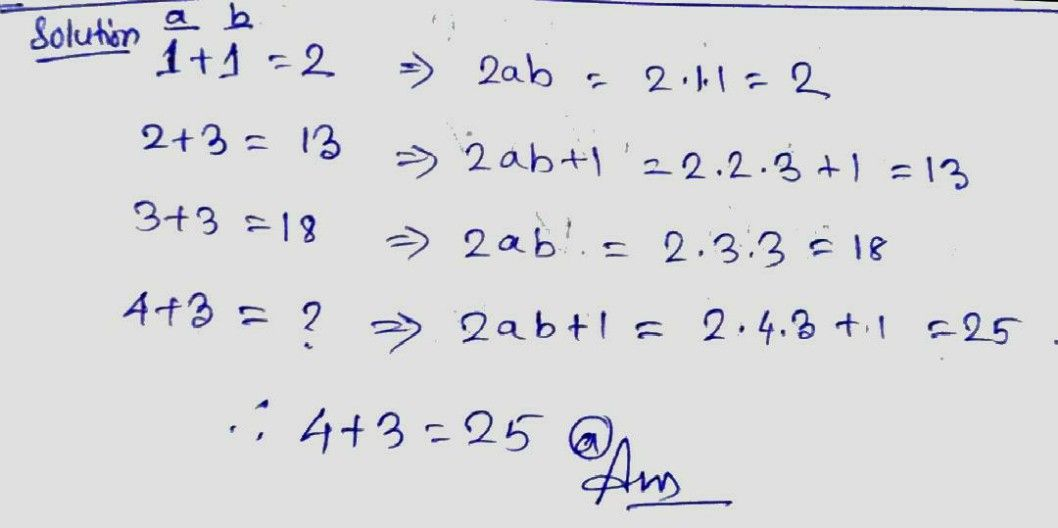Symbol
Problem$40$ If $\left(1+1=2,2+3=13,3+3=18$ them $4+3=2$ (a) $\right)$ $25$ $\left($ (b) $23$ $\left(c\right)$ $22$ $\left($ (d) $\right)$ $16$
Other
Search count: 105
SolutionQanda teacher - NehaMamIf you have any doubt feel free to ask:) Dear, Please evaluate the answer and give gift coins(it does not reduce your coin balance.) for giving gift coins Click on evaluate. Then press the + icon 3 times(3000).Student
thanks